Скачать презентацию This is a Power Point presentation on the

b5fdc89462fcef1d5afc263ff2df395a.ppt

• Количество слайдов: 44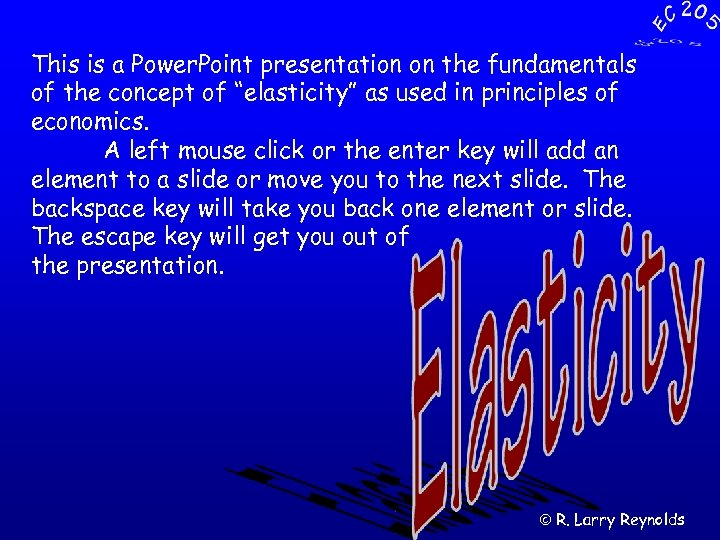This is a Power. Point presentation on the fundamentals of the concept of “elasticity” as used in principles of economics. A left mouse click or the enter key will add an element to a slide or move you to the next slide. The backspace key will take you back one element or slide. The escape key will get you out of the presentation. ã R. Larry Reynolds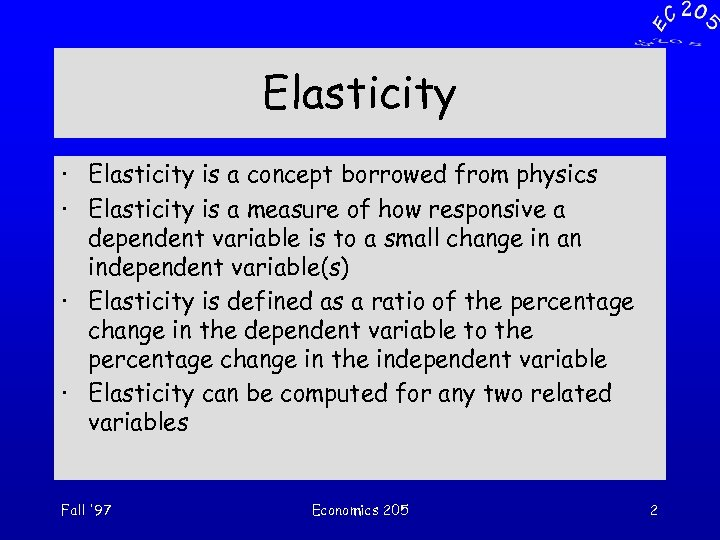Elasticity · Elasticity is a concept borrowed from physics · Elasticity is a measure of how responsive a dependent variable is to a small change in an independent variable(s) · Elasticity is defined as a ratio of the percentage change in the dependent variable to the percentage change in the independent variable · Elasticity can be computed for any two related variables Fall '97 Economics 205 2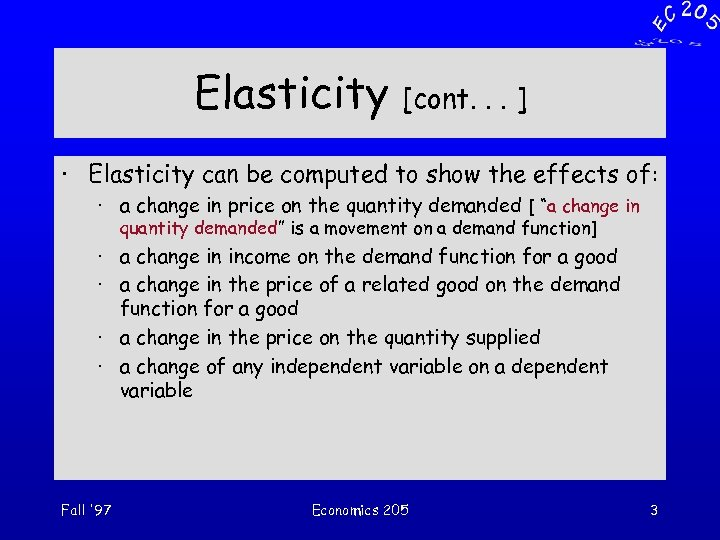Elasticity [cont. . . ] · Elasticity can be computed to show the effects of: · a change in price on the quantity demanded [ “a change in quantity demanded” is a movement on a demand function] · a change in income on the demand function for a good · a change in the price of a related good on the demand function for a good · a change in the price on the quantity supplied · a change of any independent variable on a dependent variable Fall '97 Economics 205 3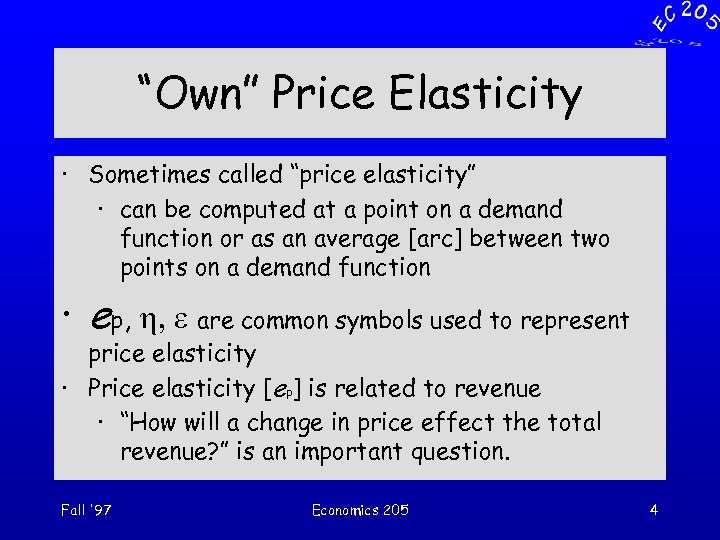“Own” Price Elasticity · Sometimes called “price elasticity” · can be computed at a point on a demand function or as an average [arc] between two points on a demand function · ep, h, e are common symbols used to represent price elasticity · Price elasticity [ep] is related to revenue · “How will a change in price effect the total revenue? ” is an important question. Fall '97 Economics 205 4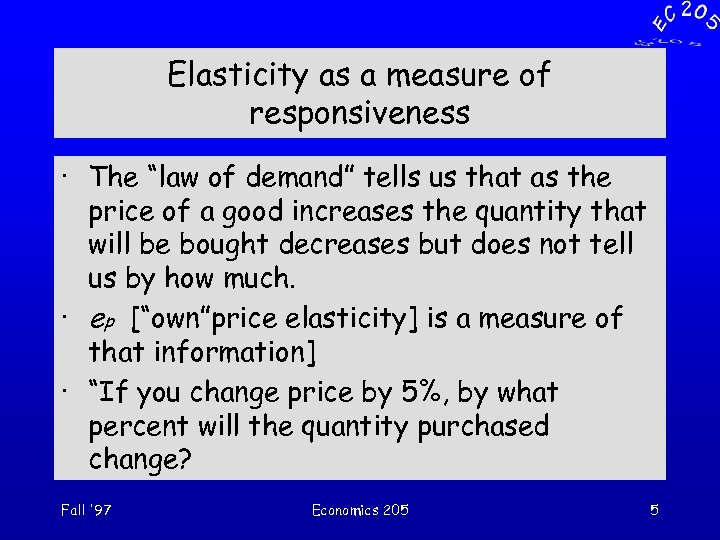Elasticity as a measure of responsiveness · The “law of demand” tells us that as the price of a good increases the quantity that will be bought decreases but does not tell us by how much. · ep [“own”price elasticity] is a measure of that information] · “If you change price by 5%, by what percent will the quantity purchased change? Fall '97 Economics 205 5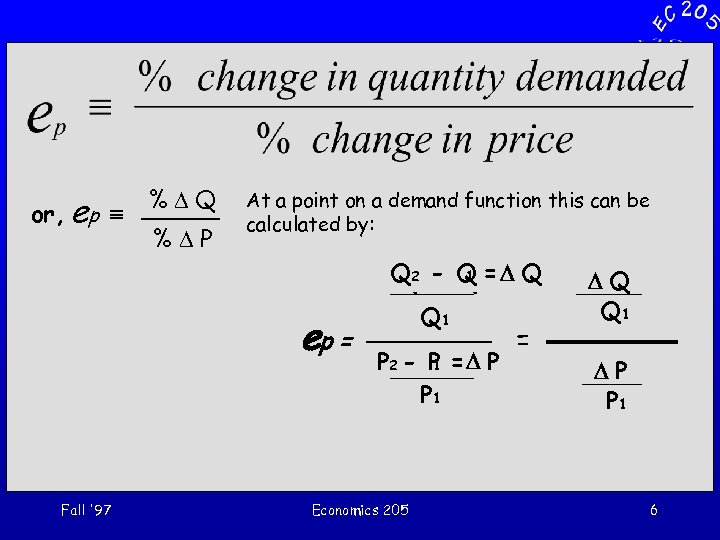or, ep º %DQ %DP At a point on a demand function this can be calculated by: ep = Fall '97 Q 22 - Q = D Q 1 Q - Q 1 P 2 P = D P - - P 1 1 P 1 Economics 205 = DQ Q 1 DP P 1 6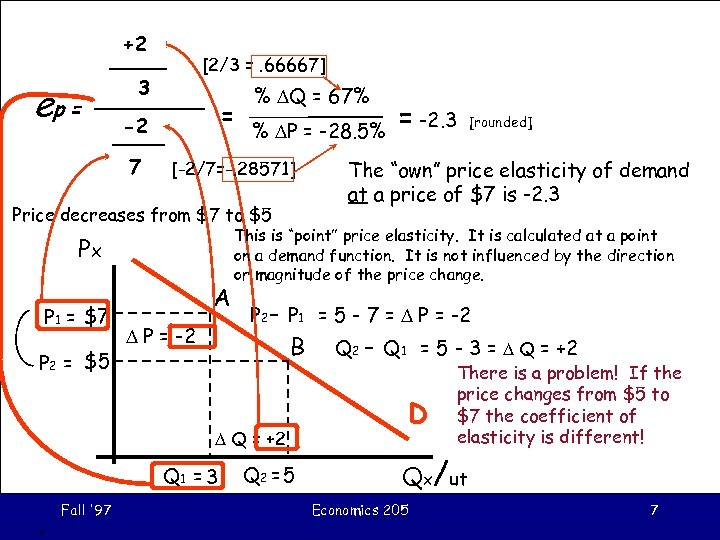+2 DQ ep = [2/3 =. 66667] 3 Q 1 = DP -2 P 1 7 % DQ = 67% % DP = -28. 5% [-2/7=-. 28571] Price decreases from \$7 to \$5 Px P 1 = \$7 P 2 = \$5 A D P = -2. 3 The “own” price elasticity of demand at a price of \$7 is -2. 3 This is “point” price elasticity. It is calculated at a point on a demand function. It is not influenced by the direction or magnitude of the price change. P 2 - P 1 = 5 - 7 = D P = -2 B Q 2 - Q 1 = 5 - 3 = D Q = +2 D D Q = +2 Q 1 = 3 . Fall '97 [rounded] Q 2 = 5 There is a problem! If the price changes from \$5 to \$7 the coefficient of elasticity is different! Qx/ut Economics 205 7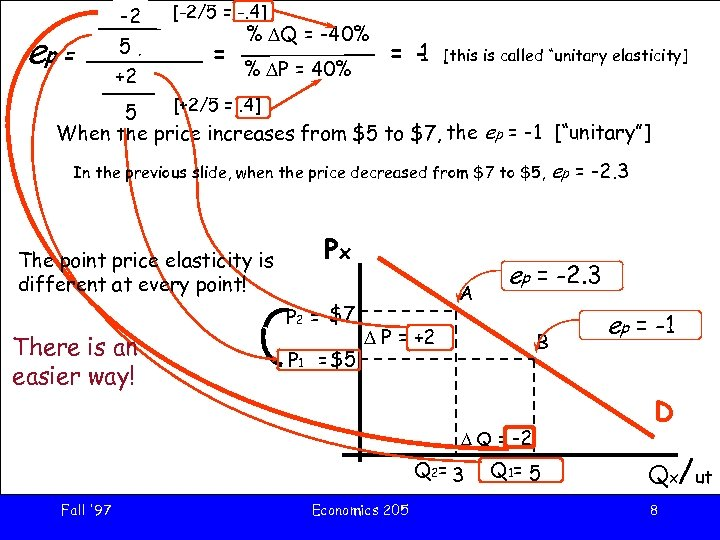ep = DQ -2 [-2/5 = -. 4] Q 5 1 = +2 DP % DQ = -40% % DP = 40% = 1 [this is called “unitary elasticity] [+2/5 =. 4] P 5 1 When the price increases from \$5 to \$7, the ep = -1 [“unitary”] In the previous slide, when the price decreased from \$7 to \$5, The point price elasticity is different at every point! There is an easier way! Px P 2 = \$7 P 1 = \$5 A ep = -2. 3 D P = +2 B D Q = -2 Q 2 = 3 Fall '97 Economics 205 ep = -2. 3 Q 1= 5 ep = -1 D Qx/ut 8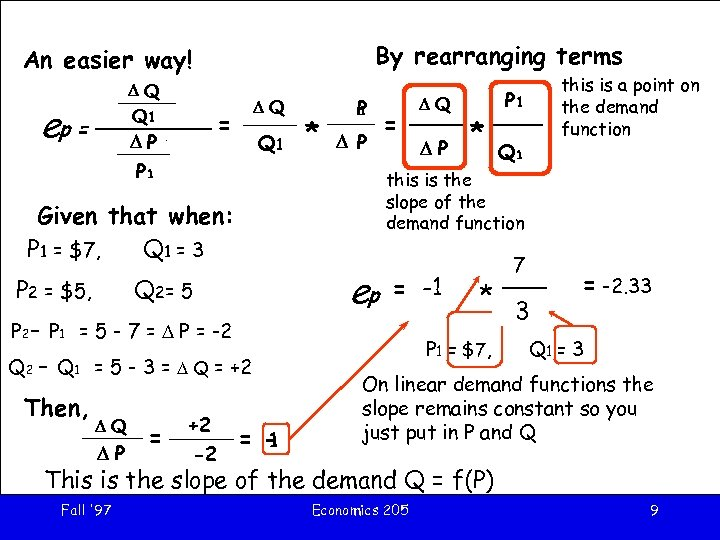By rearranging terms An easier way! DQ Q 1 D Q 1 P ep = DQ = Q 1 * P 1 D P P 1 ep Q 2= 5 P 2 - P 1 = 5 - 7 = D P = -2 Q 2 - Q 1 = 5 - 3 = D Q = +2 Then, DQ DP = +2 -2 DP * P 1 Q 1 this is the slope of the demand function Given that when: P 1 = \$7, Q 1 = 3 P 2 = \$5, = DQ this is a point on the demand function = 1 DQ = -1 DP 7 P 1 * Q 1 3 P 1 = \$7, = -2. 33 Q 1 = 3 On linear demand functions the slope remains constant so you just put in P and Q This is the slope of the demand Q = f(P) Fall '97 Economics 205 9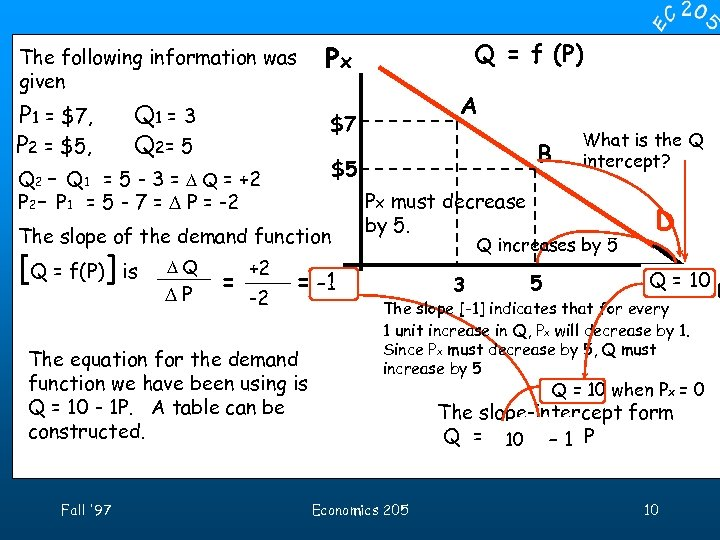The following information was given P 1 = \$7, P 2 = \$5, Q 1 = 3 Q 2= 5 DP = +2 -2 Px must decrease by 5. What is the Q intercept? Q increases by 5 = -1 The equation for the demand function we have been using is Q = 10 - 1 P. A table can be constructed. Fall '97 B \$5 The slope of the demand function DQ A \$7 Q 2 - Q 1 = 5 - 3 = D Q = +2 P 2 - P 1 = 5 - 7 = D P = -2 [Q = f(P)] is Q = f (P) Px 3 5 D / Q = 10 Qx ut The slope [-1] indicates that for every 1 unit increase in Q, Px will decrease by 1. Since Px must decrease by 5, Q must increase by 5 Q = 10 when Px = 0 The slope-intercept form Q = a 10+ - 1 P m Economics 205 10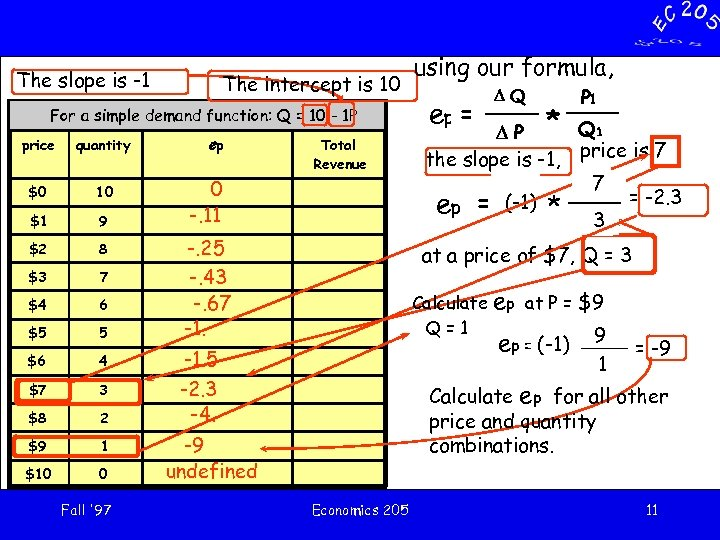The slope is -1 The intercept is 10 For a simple demand function: Q = 10 - 1 P price quantity \$0 10 \$1 9 \$2 8 \$3 7 \$4 6 \$5 5 \$6 4 \$7 3 \$8 2 \$9 1 \$10 0 Fall '97 ep Total Revenue 0 -. 11 -. 25 -. 43 -. 67 -1. using our formula, ep = DQ P 1 D P * Q 1 the slope is -1, price is 7 P 7 1 DQ ep = (-1) * Q 1 = -2. 3 3 DP at a price of \$7, Q = 3 Calculate Q=1 -1. 5 -2. 3 -4. ep at P = \$9 ep = (-1) 9 1 = -9 Calculate ep for all other price and quantity combinations. -9 undefined Economics 205 11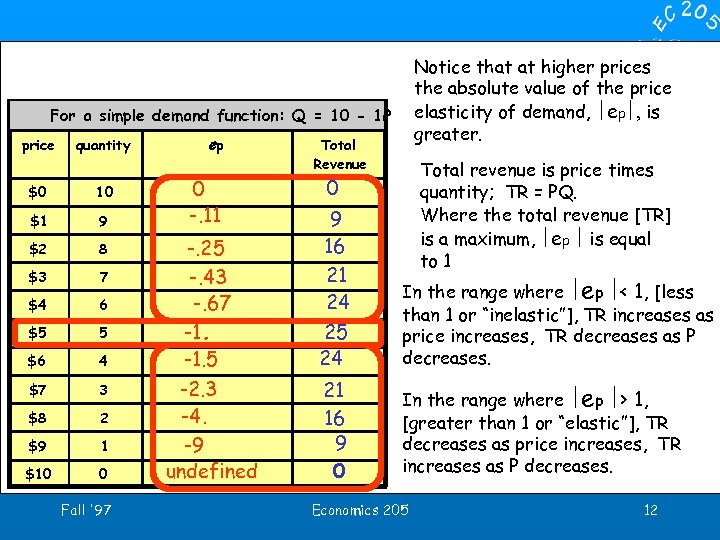Notice that at higher prices the absolute value of the price elasticity of demand, ½ep½, is greater. For a simple demand function: Q = 10 - 1 P price quantity \$0 10 \$1 9 \$2 8 \$3 7 \$4 6 \$5 5 \$6 4 \$7 3 \$8 2 \$9 1 \$10 0 Fall '97 ep 0 -. 11 -. 25 -. 43 -. 67 -1. 5 -2. 3 -4. -9 undefined Total Revenue Total revenue is price times quantity; TR = PQ. Where the total revenue [TR] is a maximum, ½ep ½ is equal to 1 0 9 16 21 24 25 24 In the range where ½ep ½< 1, [less than 1 or “inelastic”], TR increases as price increases, TR decreases as P decreases. 21 16 9 0 In the range where ½ep ½> 1, [greater than 1 or “elastic”], TR decreases as price increases, TR increases as P decreases. Economics 205 12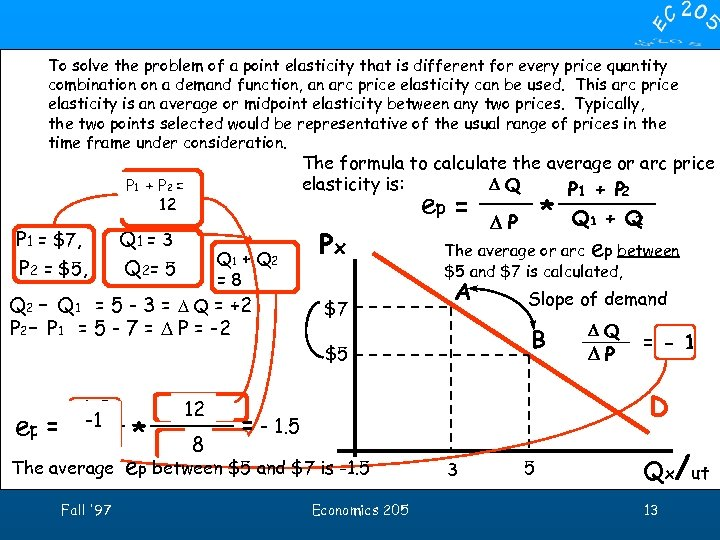To solve the problem of a point elasticity that is different for every price quantity combination on a demand function, an arc price elasticity can be used. This arc price elasticity is an average or midpoint elasticity between any two prices. Typically, the two points selected would be representative of the usual range of prices in the time frame under consideration. The formula to calculate the average or arc price elasticity is: DQ P 1 + P 2 ep = * P 1 + P 2 = 12 P 1 = \$7, P 2 = \$5, Q 1 = 3 Q 2= 5 Q 1 + Q 2 =8 Q 2 - Q 1 = 5 - 3 = D Q = +2 P 2 - P 1 = 5 - 7 = D P = -2 ep = DQ -1 DP The average Fall '97 * P 1 12 P + 2 Q 1 8 Q + 2 Px \$7 Q 1 + Q 2 DP The average or arc ep between \$5 and \$7 is calculated, A Slope of demand B \$5 DQ DP = - 1 D = - 1. 5 ep between \$5 and \$7 is -1. 5 Economics 205 3 5 Qx/ut 13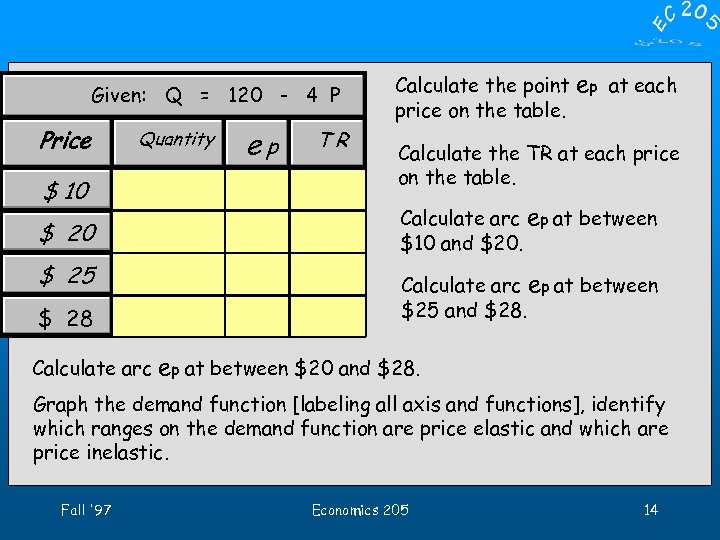Given: Q = 120 - 4 P Price Quantity \$ 10 \$ 25 \$ 28 Calculate arc ep TR Calculate the point price on the table. ep at each Calculate the TR at each price on the table. Calculate arc ep at between \$10 and \$20. Calculate arc ep at between \$25 and \$28. ep at between \$20 and \$28. Graph the demand function [labeling all axis and functions], identify which ranges on the demand function are price elastic and which are price inelastic. Fall '97 Economics 205 14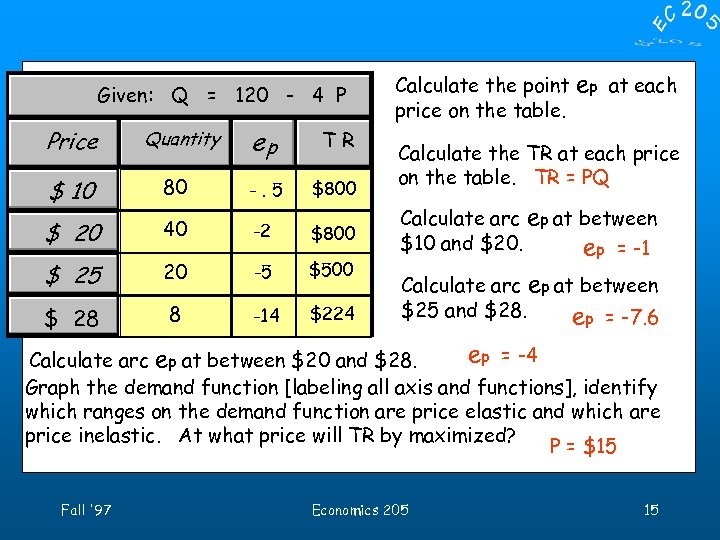Given: Q = 120 - 4 P Price Quantity ep TR \$ 10 80 -. 5 \$800 \$ 20 40 -2 \$800 \$ 25 20 -5 \$500 \$ 28 8 -14 \$224 Calculate the point price on the table. ep at each Calculate the TR at each price on the table. TR = PQ Calculate arc ep at between \$10 and \$20. ep = -1 Calculate arc ep at between \$25 and \$28. ep = -7. 6 ep = -4 Calculate arc ep at between \$20 and \$28. Graph the demand function [labeling all axis and functions], identify which ranges on the demand function are price elastic and which are price inelastic. At what price will TR by maximized? P = \$15 Fall '97 Economics 205 15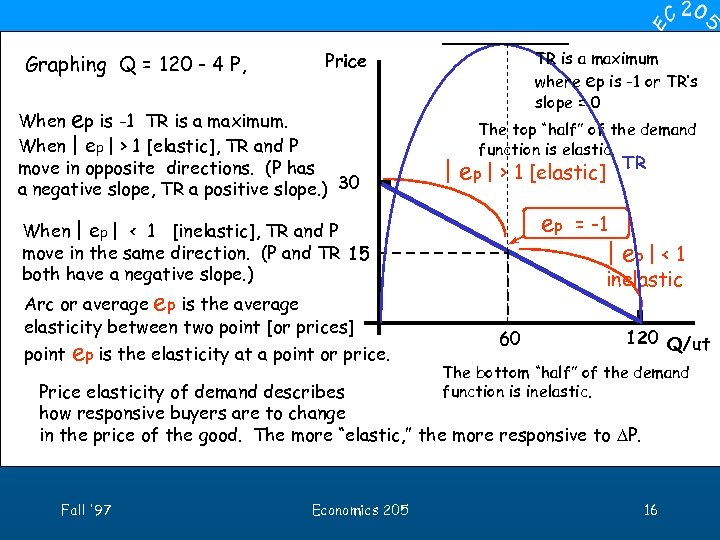Graphing Q = 120 - 4 P, TR is a maximum where ep is -1 or TR’s slope = 0 Price When ep is -1 TR is a maximum. When | ep | > 1 [elastic], TR and P move in opposite directions. (P has a negative slope, TR a positive slope. ) 30 The top “half” of the demand function is elastic. | ep | > 1 [elastic] ep = -1 | ep | < 1 When | ep | < 1 [inelastic], TR and P move in the same direction. (P and TR 15 both have a negative slope. ) Arc or average ep is the average elasticity between two point [or prices] point ep is the elasticity at a point or price. TR inelastic 60 120 Q/ut The bottom “half” of the demand function is inelastic. Price elasticity of demand describes how responsive buyers are to change in the price of the good. The more “elastic, ” the more responsive to DP. Fall '97 Economics 205 16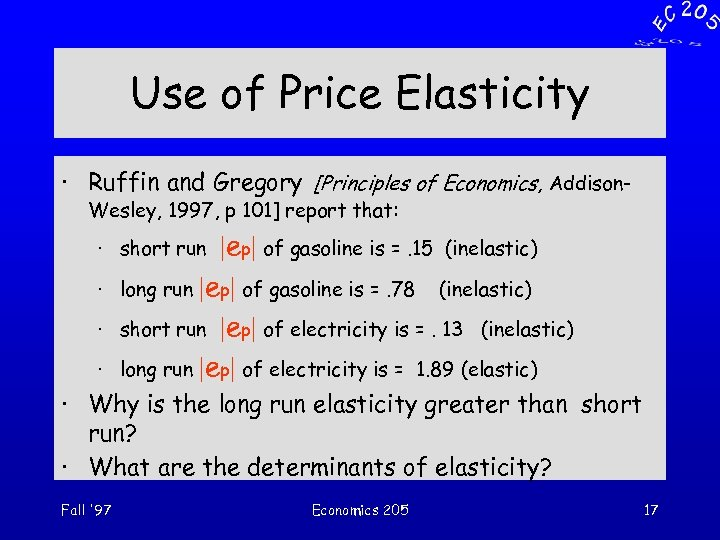Use of Price Elasticity · Ruffin and Gregory [Principles of Economics, Addison. Wesley, 1997, p 101] report that: |ep| of gasoline is =. 15 (inelastic) long run |ep| of gasoline is =. 78 (inelastic) short run |ep| of electricity is =. 13 (inelastic) long run |ep| of electricity is = 1. 89 (elastic) · short run · · Why is the long run elasticity greater than short run? · What are the determinants of elasticity? Fall '97 Economics 205 17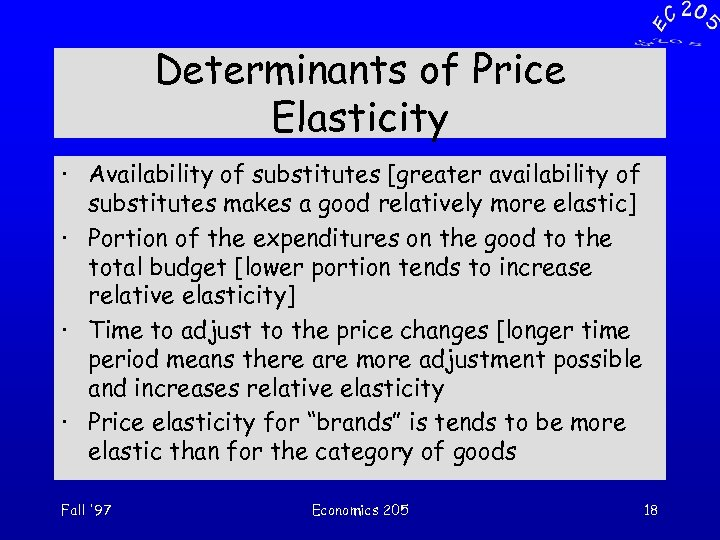Determinants of Price Elasticity · Availability of substitutes [greater availability of substitutes makes a good relatively more elastic] · Portion of the expenditures on the good to the total budget [lower portion tends to increase relative elasticity] · Time to adjust to the price changes [longer time period means there are more adjustment possible and increases relative elasticity · Price elasticity for “brands” is tends to be more elastic than for the category of goods Fall '97 Economics 205 18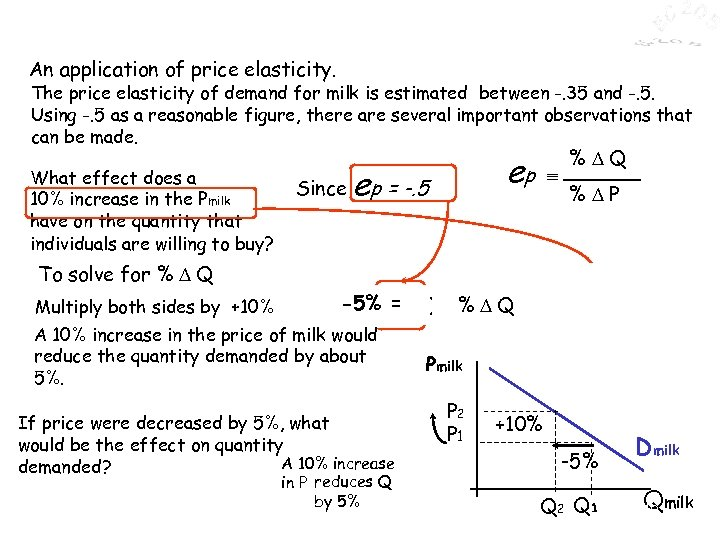An application of price elasticity. The price elasticity of demand for milk is estimated between -. 35 and -. 5. Using -. 5 as a reasonable figure, there are several important observations that can be made. What effect does a 10% increase in the Pmilk have on the quantity that individuals are willing to buy? To solve for % D Q Multiply both sides by +10% Since e -5% = p ) (+10%)x (-. 5 º A 10% increase in the price of milk would reduce the quantity demanded by about 5%. If price were decreased by 5%, what would be the effect on quantity A 10% increase demanded? Fall '97 ep ep = -. 5 in P reduces Q by 5% Economics 205 º %DQ %DP % = D Q x (+10%) %DQ % +10% DP Pmilk P 2 P 1 +10% -5% Q 2 Q 1 Dmilk Qmilk 19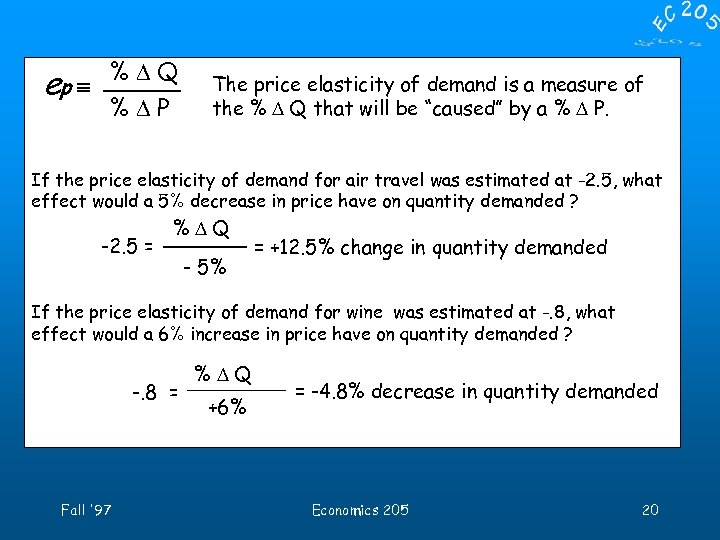%DQ ep º %DP The price elasticity of demand is a measure of the % D Q that will be “caused” by a % D P. If the price elasticity of demand for air travel was estimated at -2. 5, what effect would a 5% decrease in price have on quantity demanded ? -2. 5 = %DQ % 5%P - D = +12. 5% change in quantity demanded If the price elasticity of demand for wine was estimated at -. 8, what effect would a 6% increase in price have on quantity demanded ? -. 8 = Fall '97 %DQ %DP +6% = -4. 8% decrease in quantity demanded Economics 205 20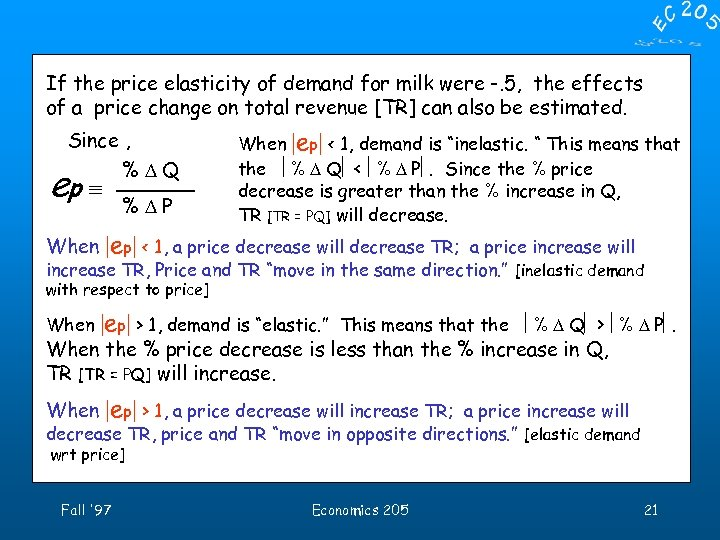If the price elasticity of demand for milk were -. 5, the effects of a price change on total revenue [TR] can also be estimated. Since , %DQ ep º When %DP When |ep| < 1, demand is “inelastic. “ This means that the ê% D Qê < ê% D Pê. Since the % price decrease is greater than the % increase in Q, TR [TR = PQ] will decrease. |ep| < 1, a price decrease will decrease TR; a price increase will increase TR, Price and TR “move in the same direction. ” [inelastic demand with respect to price] When |ep| > 1, demand is “elastic. ” This means that the ê% D Qê > ê% D Pê. When the % price decrease is less than the % increase in Q, TR [TR = PQ] will increase. When |ep| > 1, a price decrease will increase TR; a price increase will decrease TR, price and TR “move in opposite directions. ” [elastic demand wrt price] Fall '97 Economics 205 21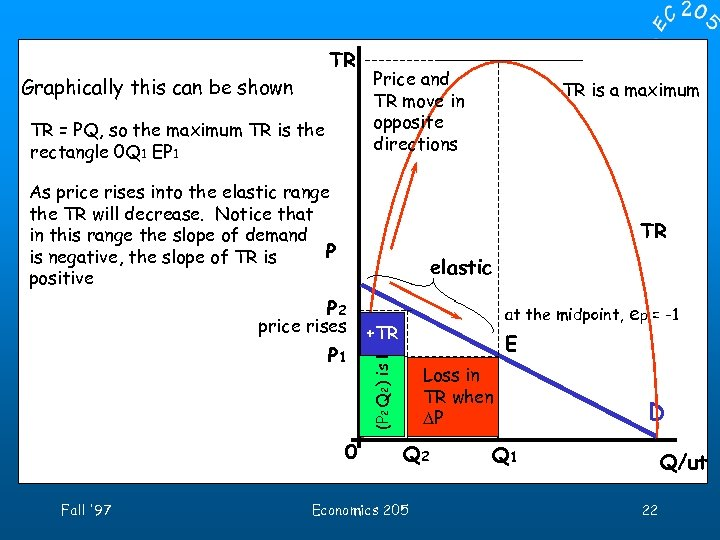TR Graphically this can be shown TR = PQ, so the maximum TR is the rectangle 0 Q 1 EP 1 Price and TR move in opposite directions As price rises into the elastic range the TR will decrease. Notice that in this range the slope of demand P is negative, the slope of TR is positive TR elastic price rises P 1 0 at the midpoint, ep = -1 +TR E (P 2 Q 2) is less P 2 Fall '97 TR is a maximum than in Loss (P 1 Q 1) TR when DP Q 2 Economics 205 D Q 1 Q/ut 22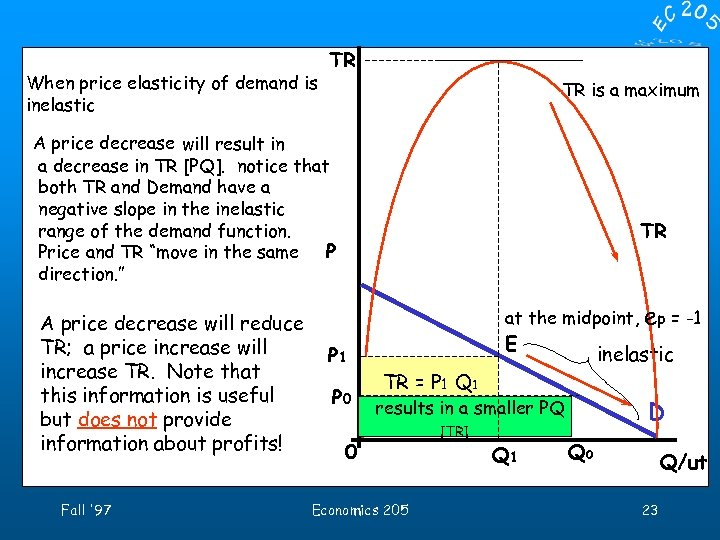When price elasticity of demand is inelastic TR TR is a maximum A price decrease will result in a decrease in TR [PQ]. notice that both TR and Demand have a negative slope in the inelastic range of the demand function. Price and TR “move in the same P direction. ” A price decrease will reduce TR; a price increase will increase TR. Note that this information is useful but does not provide information about profits! Fall '97 TR at the midpoint, P 1 P 0 E inelastic TR = P 1 Q 1 [Maximum] results in a smaller PQ 0 Economics 205 [TR] Q 1 ep = -1 D Q 0 Q/ut 23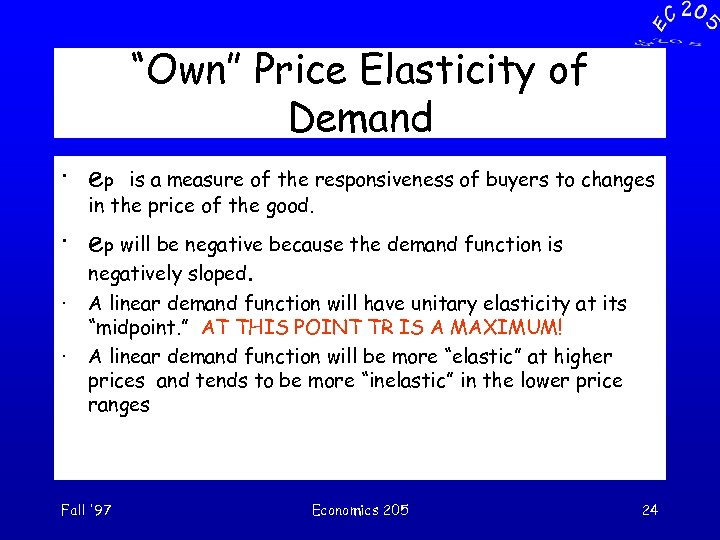“Own” Price Elasticity of Demand · ep is a measure of the responsiveness of buyers to changes in the price of the good. · ep will be negative because the demand function is · · negatively sloped. A linear demand function will have unitary elasticity at its “midpoint. ” AT THIS POINT TR IS A MAXIMUM! A linear demand function will be more “elastic” at higher prices and tends to be more “inelastic” in the lower price ranges Fall '97 Economics 205 24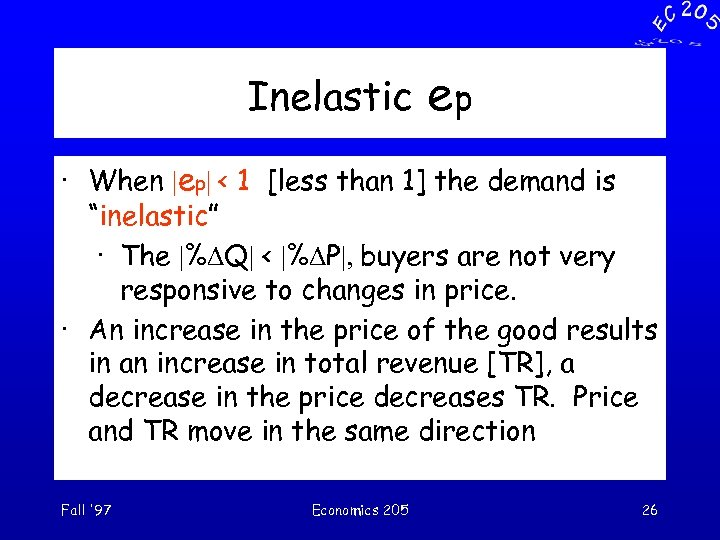Inelastic ep · When |ep| < 1 [less than 1] the demand is “inelastic” · The |%DQ| < |%DP|, buyers are not very responsive to changes in price. · An increase in the price of the good results in an increase in total revenue [TR], a decrease in the price decreases TR. Price and TR move in the same direction Fall '97 Economics 205 26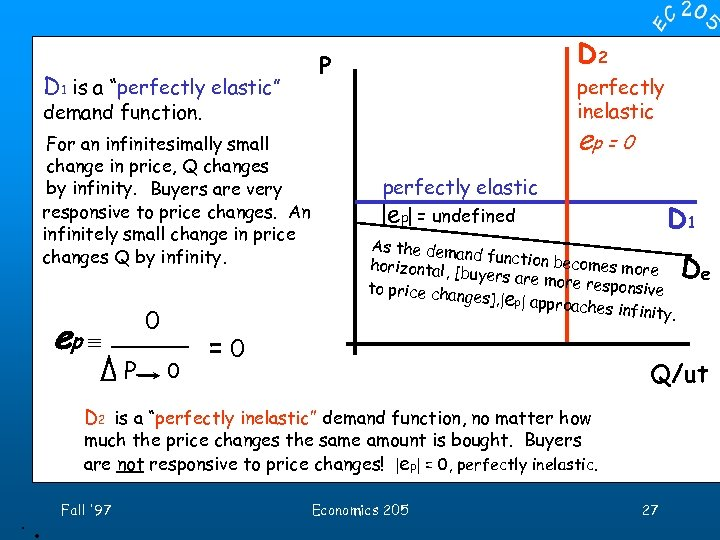D 2 P D 1 is a “perfectly elastic” perfectly inelastic demand function. ep = 0 For an infinitesimally small change in price, Q changes by infinity. Buyers are very responsive to price changes. An infinitely small change in price changes Q by infinity. ep º 0 %¥Q D %DP P 0 perfectly elastic |ep| = undefined D 1 As the dema nd function b ecomes more horizontal, [b uyers are mo re responsive to price chan ges], |ep| appr oaches infinit y. = undefined = 0¥ Q/ut D 2 is a “perfectly inelastic” demand function, no matter how much the price changes the same amount is bought. Buyers are not responsive to price changes! |ep| = 0, perfectly inelastic. . . Fall '97 Economics 205 De 27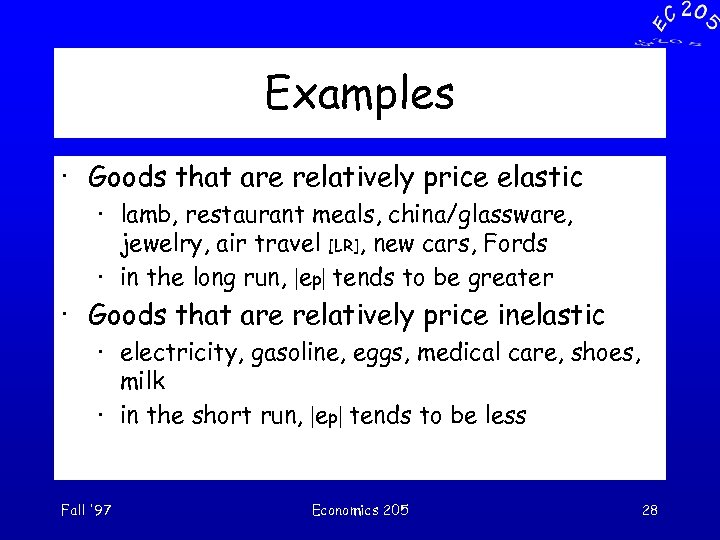Examples · Goods that are relatively price elastic · lamb, restaurant meals, china/glassware, jewelry, air travel [LR], new cars, Fords · in the long run, |ep| tends to be greater · Goods that are relatively price inelastic · electricity, gasoline, eggs, medical care, shoes, milk · in the short run, |ep| tends to be less Fall '97 Economics 205 28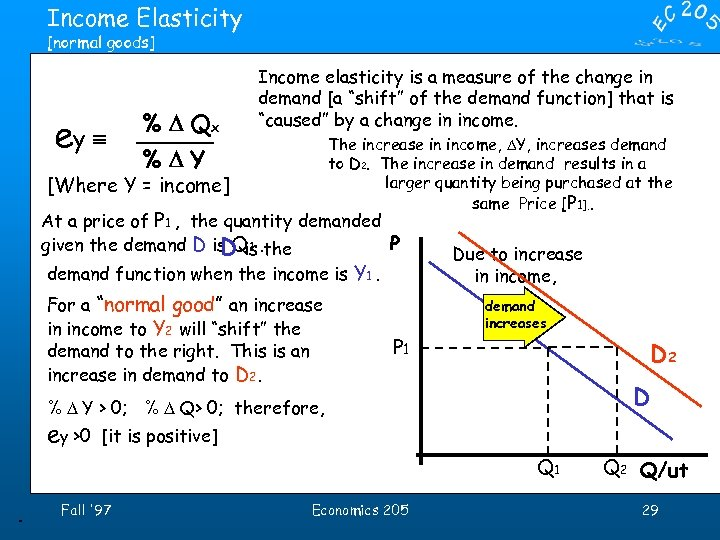Income Elasticity [normal goods] ey º % D Qx Income elasticity is a measure of the change in demand [a “shift” of the demand function] that is “caused” by a change in income. The increase in income, DY, increases demand to D 2. The increase in demand results in a larger quantity being purchased at the same Price [P 1]. . %DY [Where Y = income] At a price of P 1 , the quantity demanded P given the demand D is Q 1. the D is demand function when the income is Y 1. For a “normal good” an increase in income to Y 2 will “shift” the demand to the right. This is an increase in demand to D 2. Due to increase in income, demand increases P 1 D 2 D % D Y > 0; % D Q> 0; therefore, ey >0 [it is positive] Q 1. Fall '97 Economics 205 Q 2 Q/ut 29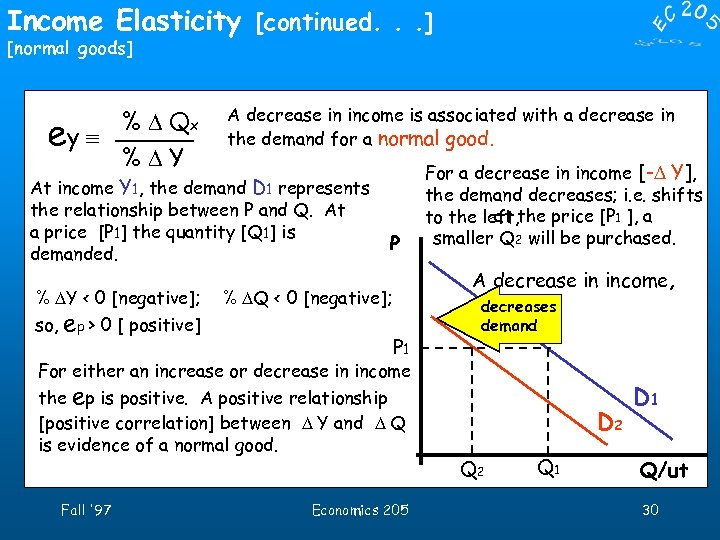Income Elasticity [continued. . . ] [normal goods] % D Qx ey º %DY A decrease in income is associated with a decrease in the demand for a normal good. At income Y 1, the demand D 1 represents the relationship between P and Q. At a price [P 1] the quantity [Q 1] is demanded. % DY < 0 [negative]; so, ep > 0 [ positive] P For a decrease in income [-D Y], the demand decreases; i. e. shifts at to the left, the price [P 1 ], a smaller Q 2 will be purchased. A decrease in income, % DQ < 0 [negative]; P 1 decreases demand For either an increase or decrease in income the ep is positive. A positive relationship [positive correlation] between D Y and D Q is evidence of a normal good. Fall '97 Economics 205 D 2 Q 1 D 1 Q/ut 30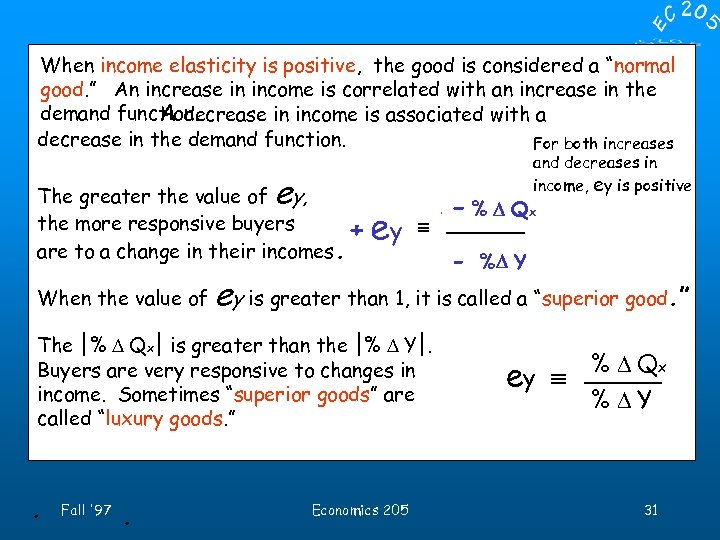When income elasticity is positive, the good is considered a “normal good. ” An increase in income is correlated with an increase in the demand function. A decrease in income is associated with a decrease in the demand function. For both increases e The greater the value of y, the more responsive buyers + are to a change in their incomes. When the value of e eyy º Fall '97 . %D DQ +- % Qxx x %DQ %DY +- %% DY D Y ey is greater than 1, it is called a “superior good. ” The |% D Qx| is greater than the |% D Y|. Buyers are very responsive to changes in income. Sometimes “superior goods” are called “luxury goods. ” . and decreases in income, ey is positive Economics 205 ey º % D Qx %DY 31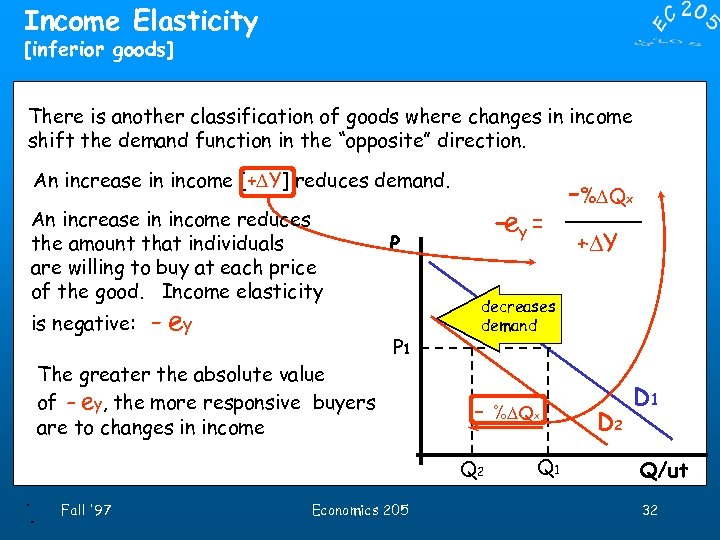Income Elasticity [inferior goods] There is another classification of goods where changes in income shift the demand function in the “opposite” direction. An increase in income [+DY] reduces demand. An increase in income reduces the amount that individuals are willing to buy at each price of the good. Income elasticity is negative: - ey The greater the absolute value of - ey, the more responsive buyers are to changes in income ey = -ey º P P 1 . Fall '97 Economics 205 x x %DY +DY decreases demand - %DQ Q 2 . -%DQ x Q 1 D 2 D 1 Q/ut 32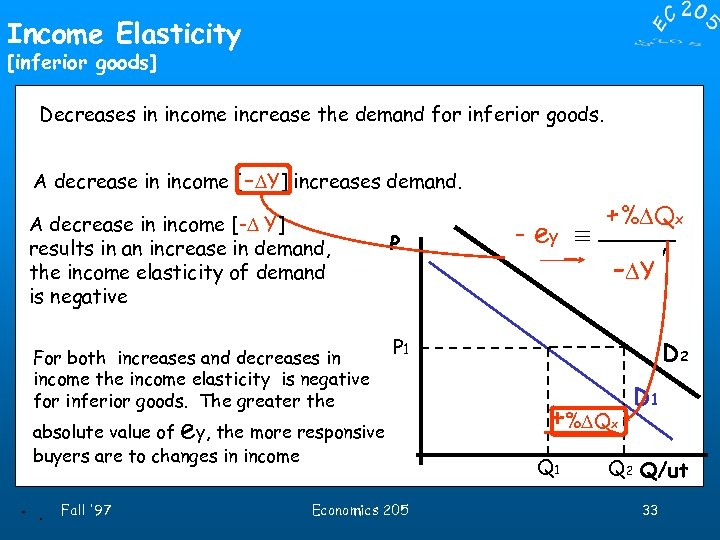Income Elasticity [inferior goods] Decreases in income increase the demand for inferior goods. A decrease in income [-DY] increases demand. A decrease in income [-D Y] results in an increase in demand, the income elasticity of demand is negative For both increases and decreases in income the income elasticity is negative for inferior goods. The greater the P P 1 absolute value of ey, the more responsive buyers are to changes in income. . Fall '97 + D Qx %%DQx - ey y º e %DY -DY Economics 205 D 2 +%DQ Q 1 D 1 x Q 2 Q/ut 33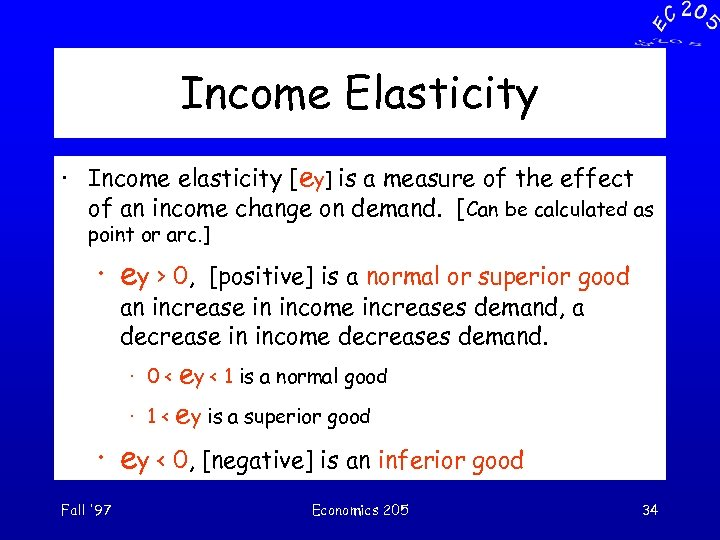Income Elasticity · Income elasticity [ey] is a measure of the effect of an income change on demand. [Can be calculated as point or arc. ] · ey > 0, [positive] is a normal or superior good an increase in income increases demand, a decrease in income decreases demand. · 0 < ey < 1 is a normal good · 1 < ey is a superior good · ey < 0, [negative] is an inferior good Fall '97 Economics 205 34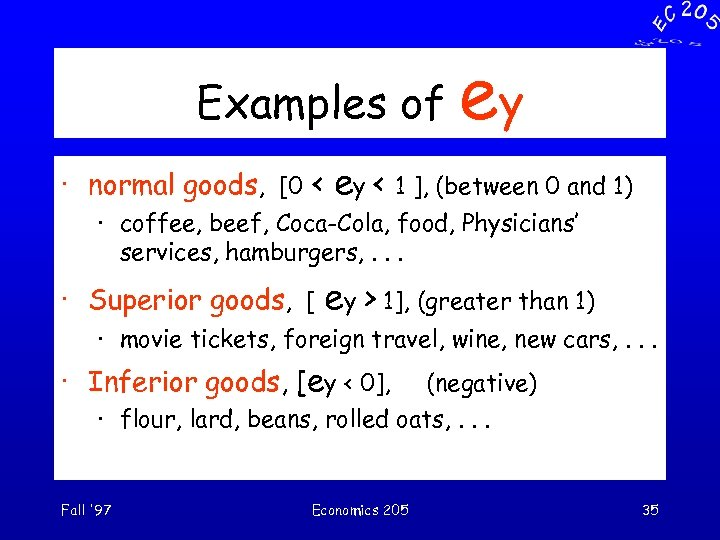Examples of · normal goods, [0 < ey ey < 1 ], (between 0 and 1) · coffee, beef, Coca-Cola, food, Physicians’ services, hamburgers, . . . · Superior goods, [ ey > 1], (greater than 1) · movie tickets, foreign travel, wine, new cars, . . . · Inferior goods, [ey < 0], (negative) · flour, lard, beans, rolled oats, . . . Fall '97 Economics 205 35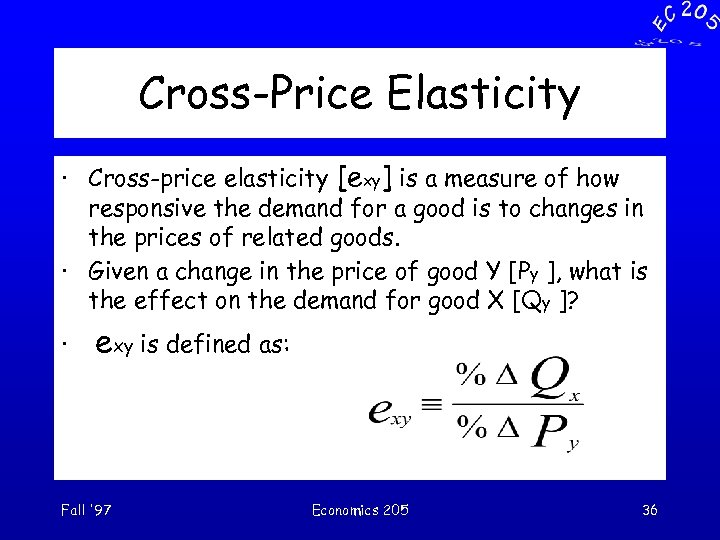Cross-Price Elasticity · Cross-price elasticity [exy] is a measure of how responsive the demand for a good is to changes in the prices of related goods. · Given a change in the price of good Y [Py ], what is the effect on the demand for good X [Qy ]? · exy is defined as: Fall '97 Economics 205 36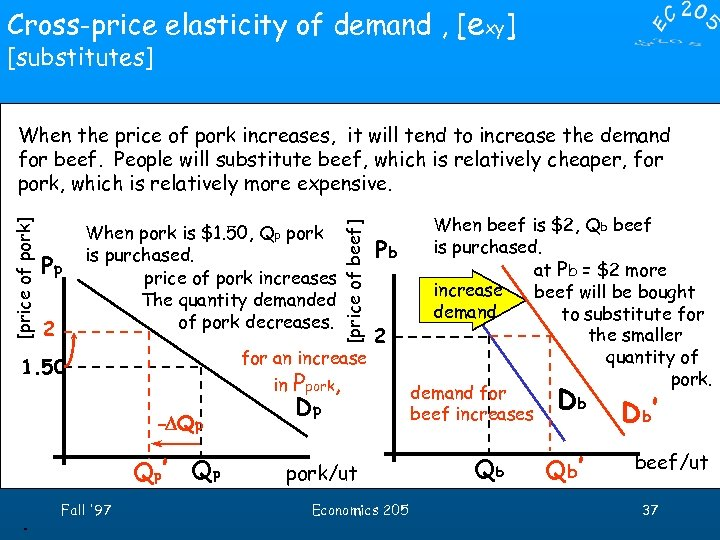Cross-price elasticity of demand , [exy] [substitutes] Pp 2 1. 50 -DQp Q p’ Q p. When beef is \$2, Qb beef Pb is purchased. at Pb = \$2 more increase beef will be bought demand to substitute for the smaller 2 quantity of for an increase pork. in Ppork, demand for Db Db Dp beef increases When pork is \$1. 50, Qp pork is purchased. price of pork increases The quantity demanded of pork decreases. Fall '97 [price of beef] [price of pork] When the price of pork increases, it will tend to increase the demand for beef. People will substitute beef, which is relatively cheaper, for pork, which is relatively more expensive. ’ pork/ut Economics 205 Qb Q b’ beef/ut 37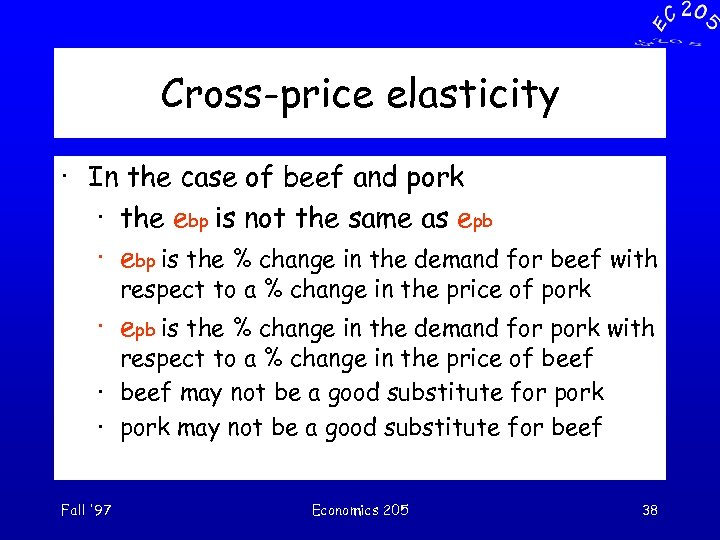Cross-price elasticity · In the case of beef and pork · the ebp is not the same as epb · ebp is the % change in the demand for beef with respect to a % change in the price of pork · epb is the % change in the demand for pork with respect to a % change in the price of beef · beef may not be a good substitute for pork · pork may not be a good substitute for beef Fall '97 Economics 205 38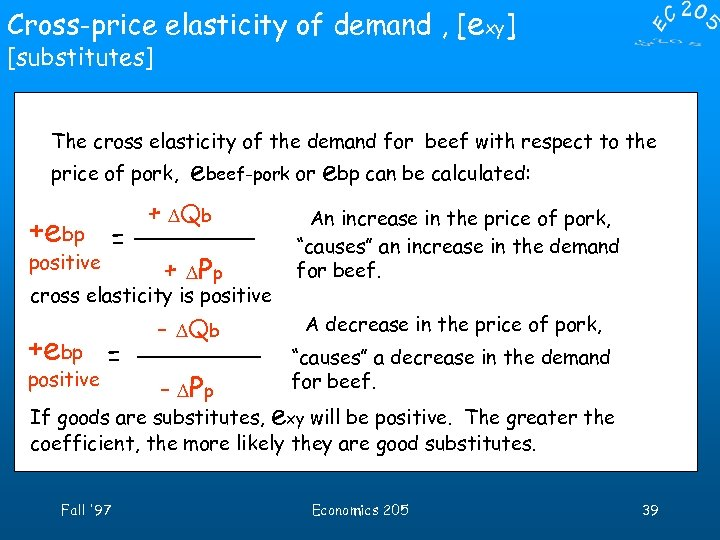Cross-price elasticity of demand , [exy] [substitutes] The cross elasticity of the demand for beef with respect to the price of pork, +ebpbp = e positive ebeef-pork or ebp can be calculated: + D of %D QQb beef %DP of Pp + D pork cross elasticity is positive +eebp = bp positive %D- DQbeef Q of b %DP of pork - DPp An increase in the price of pork, “causes” an increase in the demand for beef. A decrease in the price of pork, “causes” a decrease in the demand for beef. If goods are substitutes, exy will be positive. The greater the coefficient, the more likely they are good substitutes. Fall '97 Economics 205 39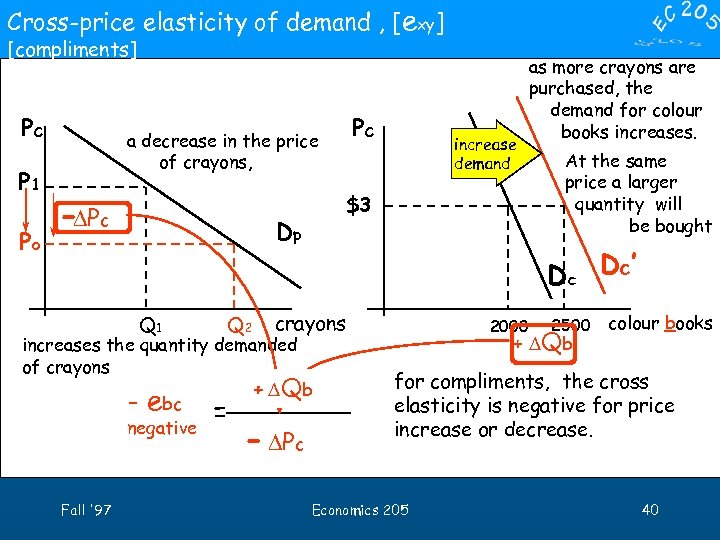Cross-price elasticity of demand , [exy] [compliments] Pc P 1 Po Pc a decrease in the price of crayons, -DPc \$3 Dp Q 1 Q 2 negative crayons Fall '97 + D Qb %D Q of b - DPc At the same price a larger quantity will be bought D c’ increases the quantity demanded of crayons - ebcbc = e increase demand as more crayons are purchased, the demand for colour books increases. %DP of c 2000 2500 + D Qb colour books for compliments, the cross elasticity is negative for price increase or decrease. Economics 205 40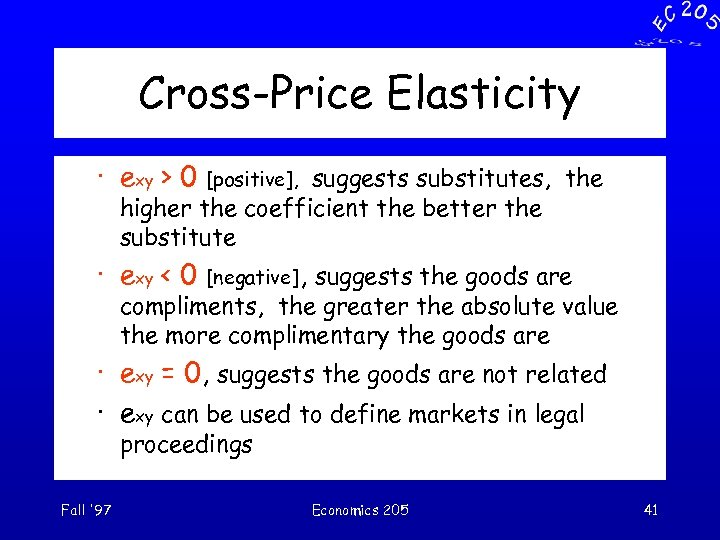Cross-Price Elasticity · exy > 0 [positive], suggests substitutes, the higher the coefficient the better the substitute · exy < 0 [negative], suggests the goods are compliments, the greater the absolute value the more complimentary the goods are · exy = 0, suggests the goods are not related · exy can be used to define markets in legal proceedings Fall '97 Economics 205 41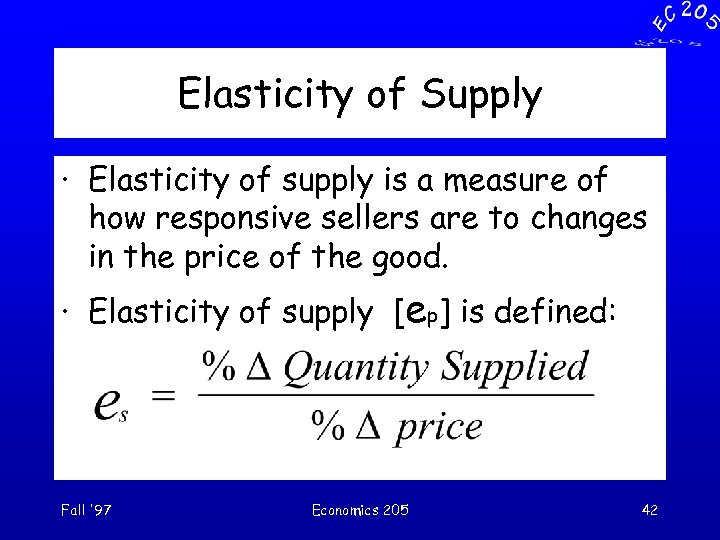Elasticity of Supply · Elasticity of supply is a measure of how responsive sellers are to changes in the price of the good. · Elasticity of supply [ep] is defined: Fall '97 Economics 205 42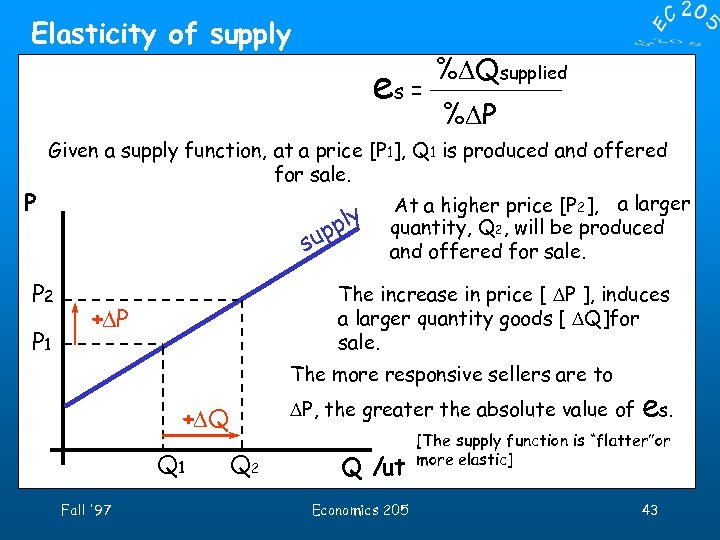Elasticity of supply es = P %DQsupplied %DP Given a supply function, at a price [P 1], Q 1 is produced and offered for sale. a larger At a higher [P y quantity, Q 2, pricebe 2], l will produced pp u s and offered for sale. P 2 P 1 The increase in price [ DP ], induces a larger quantity goods [ DQ]for sale. +DP The more responsive sellers are to DP, the greater the absolute value of +DQ Q 1 Fall '97 Q 2 Q /ut Economics 205 e s. [The supply function is “flatter”or more elastic] 43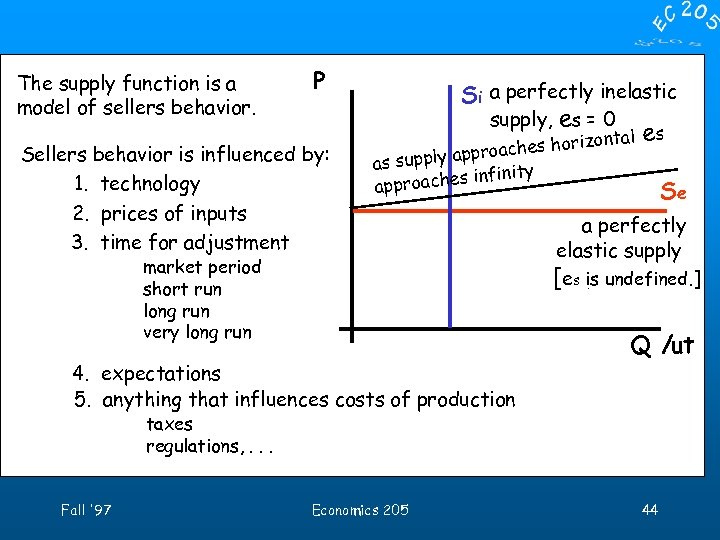The supply function is a model of sellers behavior. P Sellers behavior is influenced by: 1. technology 2. prices of inputs 3. time for adjustment Si a perfectly inelastic supply, es = 0 es horizontal s oache supply appr as infinity pproaches a market period short run long run very long run 4. expectations 5. anything that influences costs of production Se a perfectly elastic supply [es is undefined. ] Q /ut taxes regulations, . . . Fall '97 Economics 205 44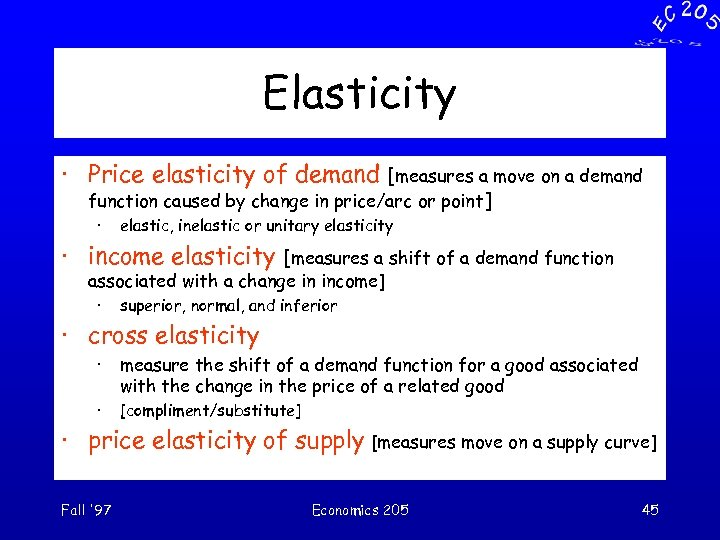Elasticity · Price elasticity of demand [measures a move on a demand function caused by change in price/arc or point] · elastic, inelastic or unitary elasticity · income elasticity [measures a shift of a demand function associated with a change in income] · superior, normal, and inferior · cross elasticity · measure the shift of a demand function for a good associated with the change in the price of a related good · [compliment/substitute] · price elasticity of supply [measures move on a supply curve] Fall '97 Economics 205 45